All TutorialsCoursesEvents & ContestsModule

Take Input in JavaScript

Print in JavaScript

Variables in JavaScript

Data Types in JavaScript

Take Input in JavaScript

# Take Input in JavaScript

By Mukul Latiyan

Updated on : 14 Oct 2022

Published on : 14 Oct 2022

## Introduction

There are often many occurrences when we want to work with some data, and the data can be made available by the user inputting them. Hence, it's important to understand how we can take inputs in JavaScript.

By default, there is one method that we can use if we want to take user input in javascript, and that is the prompt() function of the window object.

Let's make use of the prompt() function to understand it better.

## prompt()

The prompt() function is used to tell the browser to display a dialog with an optional message. Like say, we want to prompt the user with a message and expect them to enter some details about them.

The syntax of the prompt() method is shown below.

Syntax:
```.css-135pvw4{margin-top:10px;margin-bottom:10px;width:100%;}```prompt()
prompt(msg)
prompt(msg, defaultValue)
``````

The parameters that we pass in the prompt() method are

msg - A text that we want to display to the user. We can omit this, too, if we don't want to show anything to the user.

defaultValue - A text that contains the default value that will be displayed in the text input field. And in earlier versions of Internet Explorers, the string "undefined" is the default value that will be provided to the input text field.

index.html
``````<!DOCTYPE html>
<html lang="en">

<meta charset="UTF-8">
<meta http-equiv="X-UA-Compatible" content="IE=edge">
<meta name="viewport" content="width=device-width, initial-scale=1.0">
<title>Data Types in JavaScript</title>

<body>
<h1>prompt - Example</h1>

<script>
</script>
</body>

</html>
``````

Explanation - In the above example, we are creating a simple prompt where we are asking the user to enter some value in the input field.

If we run the above code in the browser, we will get the following output.

Output: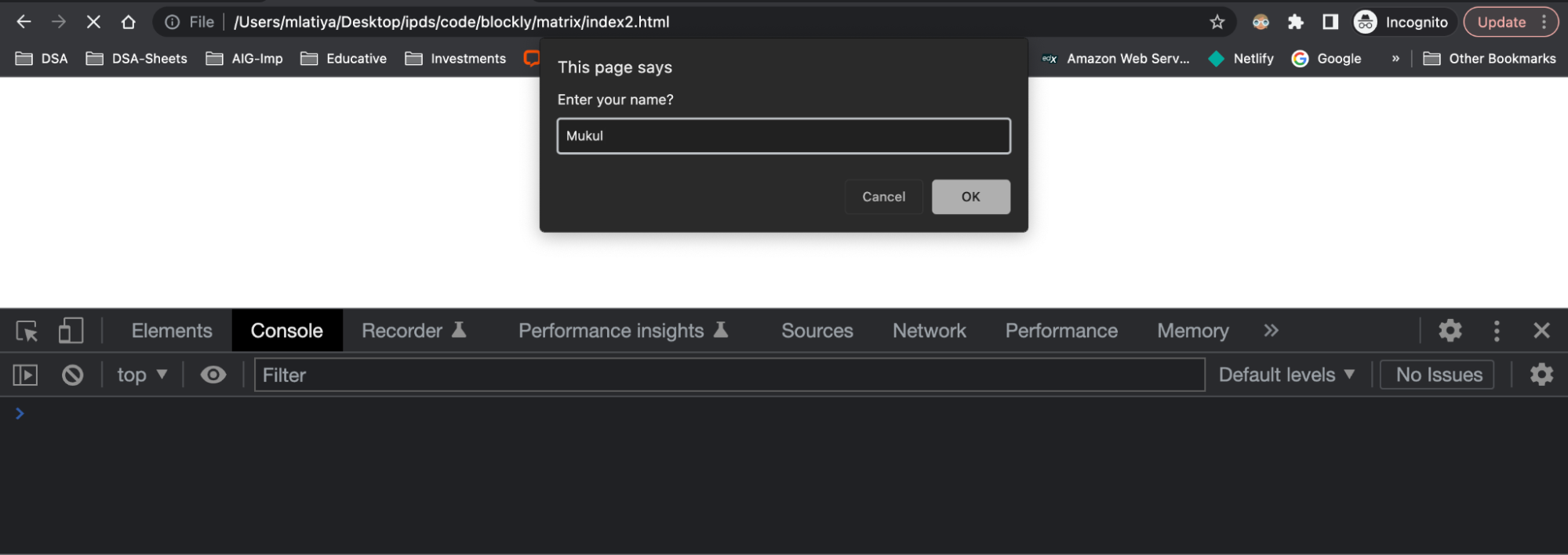In the above code, we have asked the user for some input, but we didn't do anything with that user's input. In the next example, we will explore how we can make use of text that the user inputs and store it in a variable.

index.html
``````<!DOCTYPE html>
<html lang="en">

<meta charset="UTF-8">
<meta http-equiv="X-UA-Compatible" content="IE=edge">
<meta name="viewport" content="width=device-width, initial-scale=1.0">
<title>Data Types in JavaScript</title>

<body>
<h1>prompt - Example - 1</h1>

<script>
let name = prompt('Enter your name?');

console.log(name);
</script>
</body>

</html>
``````

Explanation -

In the above code, we are creating a simple prompt where we are asking the user to enter his/her name.

We have then assigned the input value to the name variable and printed the variable.

If we run the above code in the browser, we will get the following output.

Output:
The first image below depicts the initial output before the user enters the value in the prompt's text input.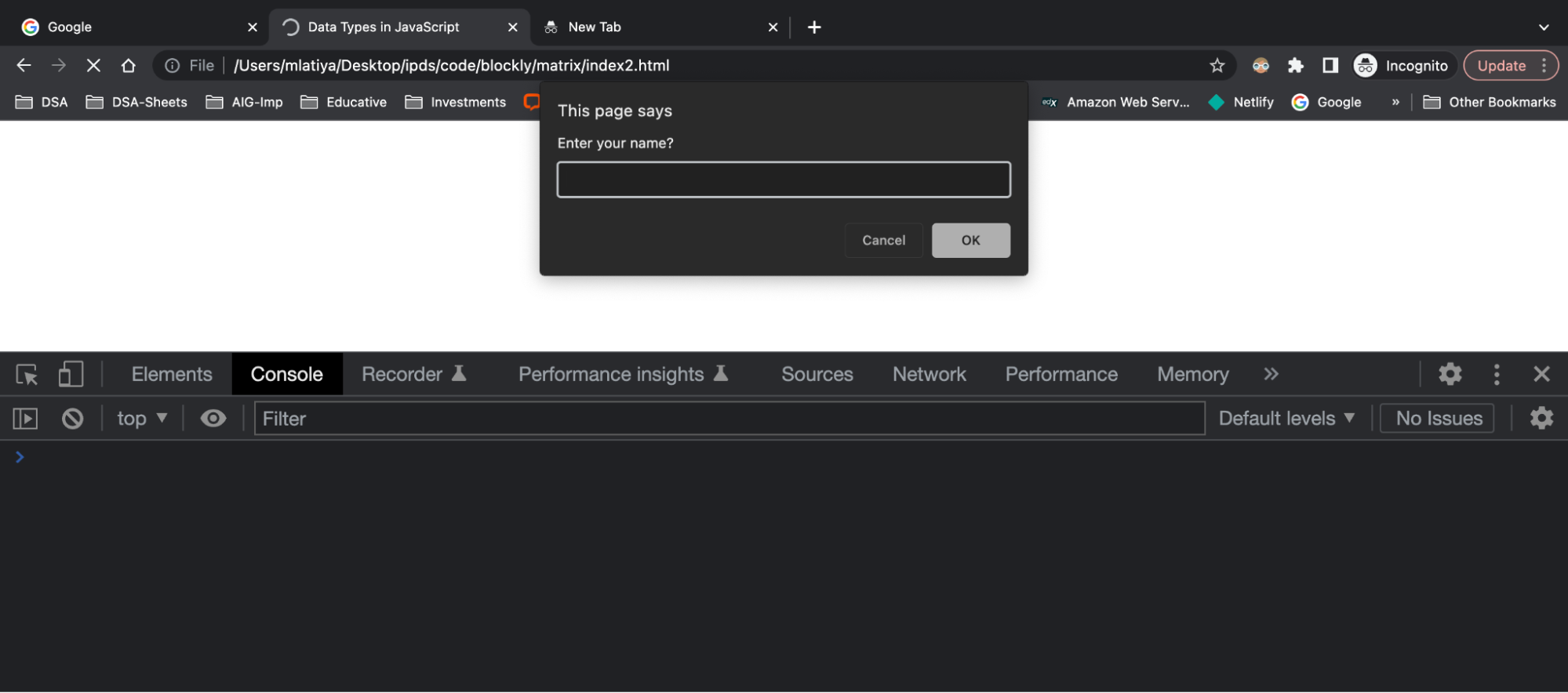Once we enter the value in the prompt's text input, we will get the following output in the browser console.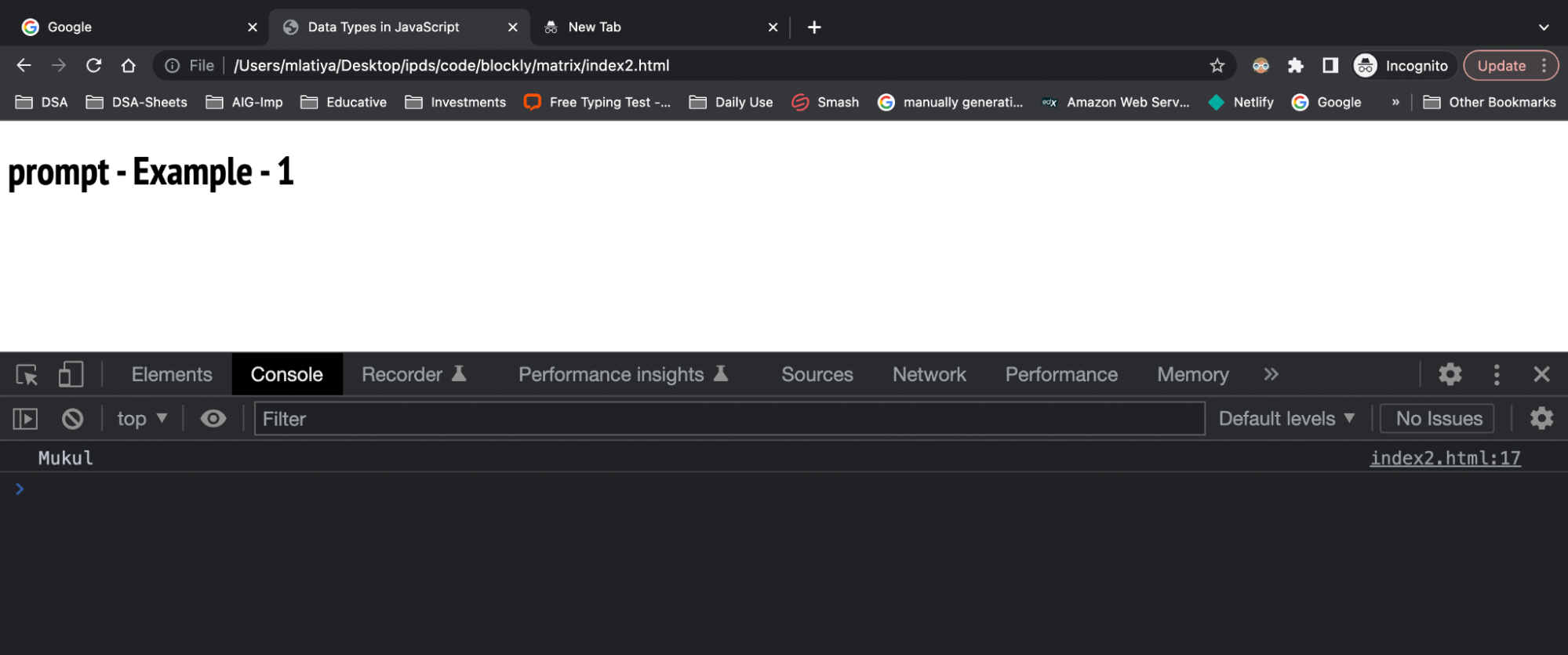## Editing DOM Element with Input Value using Prompt

In the above example, we received the input value using the prompt() function and printed the result. Now we will use the input value to change the HTML element.

Consider the code shown below.

index.html
``````<!DOCTYPE html>
<html lang="en">

<meta charset="UTF-8">
<meta http-equiv="X-UA-Compatible" content="IE=edge">
<meta name="viewport" content="width=device-width, initial-scale=1.0">
<title>Data Types in JavaScript</title>

<body>
<h1>prompt - Example - 2</h1>

<h2 id="prompt"></h2>

<script>
// asking user to enter his/her name
let name = prompt('Enter your name?');

// changing the element with id = prompt.
document.getElementById('prompt').innerHTML = name;
</script>
</body>

</html>
``````

Explanation - In the above example, we are creating a simple prompt where we are asking the user to enter his/her name.

We have used the getElementById method of the document object to modify the HTML element with id "prompt". Here, we are assigning the input value name to this HTML element.

If we run the above code in the browser, we will get the following output.

Output:
At first, we will get the following output in the browser.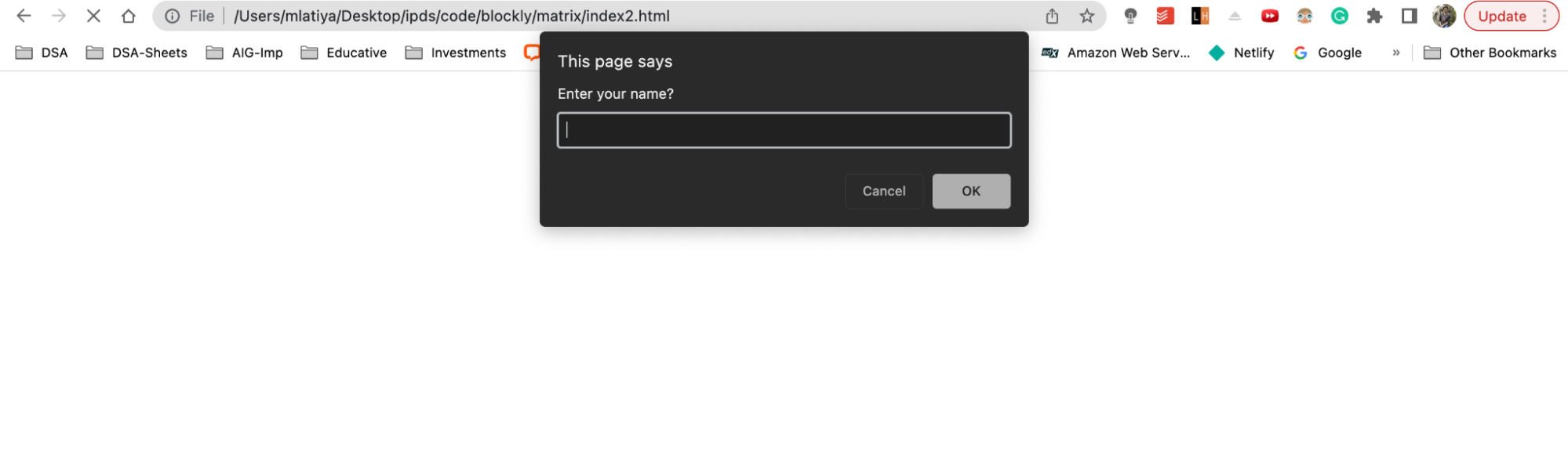Now, once we enter the value in the above prompt, we will get that input value displayed as shown below in the image.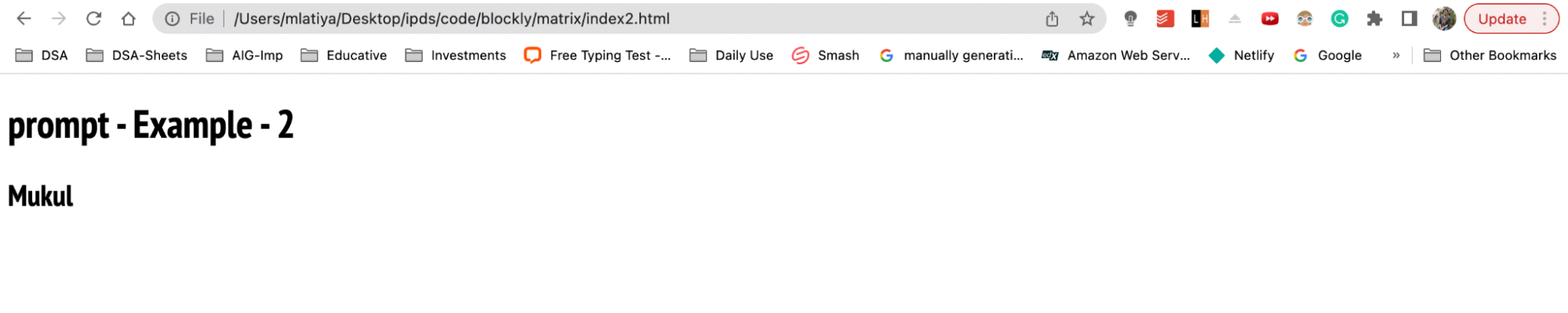## prompt() with Default Value

As mentioned before, we can also provide a default value along with the prompt() function. If provided, the default value is shown in the text box and if users don't provide any values, then the default value is used.

Let's see an example,
``````
<!DOCTYPE html>
<html lang="en">

<meta charset="UTF-8">
<meta http-equiv="X-UA-Compatible" content="IE=edge">
<meta name="viewport" content="width=device-width, initial-scale=1.0">
<title>Data Types in JavaScript</title>

<body>
<h1>prompt - Example - 1</h1>

<script>
let name = prompt('Enter your name?', 'Default Name');

console.log(name);
</script>
</body>

</html>

``````

In the above code, you can see the prompt() function now has two values: 'Enter your name?' (message to the user) and 'Default Name' (a default value).

After running the code, we will see the prompt to enter the name.

Output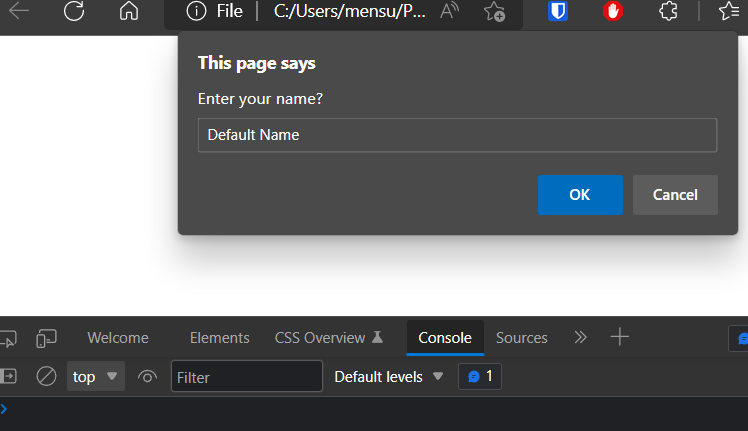If we don't provide any value and press OK, we will see the following output in the console.

Output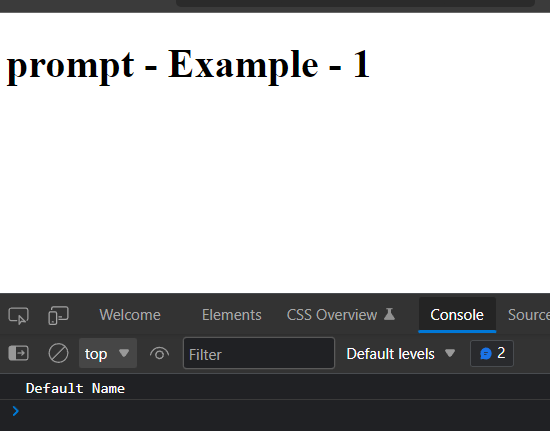## Conclusion

In this article, we learned how we could take user input in JavaScript with the help of the prompt(). We also explored examples of using the input value and getting the default value as input.## Check Out Programs Offered By Masai

If you are currently studying in 1st, 2nd or pre-final year of college,

Connect with a growingcommunity of learners